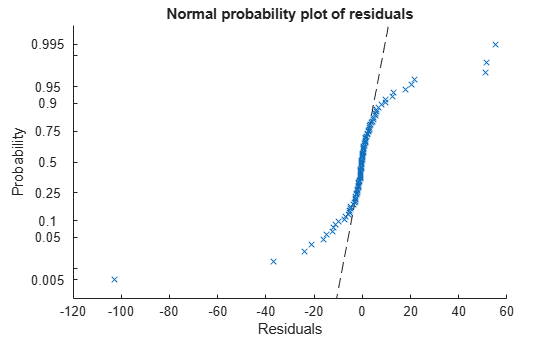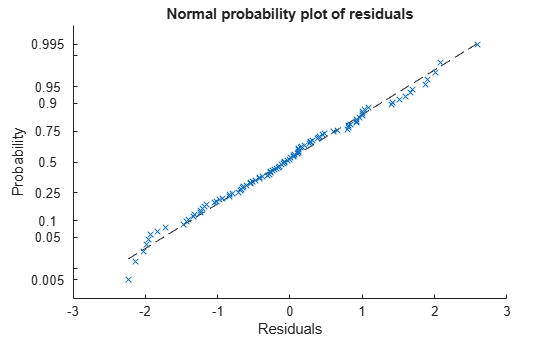# plotResiduals

Plot residuals of generalized linear regression model

## Syntax

``plotResiduals(mdl)``
``plotResiduals(mdl,plottype)``
``plotResiduals(mdl,plottype,Name,Value)``
``h = plotResiduals(___)``

## Description

example

````plotResiduals(mdl)` creates a histogram plot of the generalized linear regression model (`mdl`) residuals.```

example

````plotResiduals(mdl,plottype)` specifies the residual plot type `plottype`.```

example

````plotResiduals(mdl,plottype,Name,Value)` specifies additional options using one or more name-value pair arguments. For example, you can specify the residual type and the graphical properties of residual data points.```
````h = plotResiduals(___)` returns graphics objects for the lines or patch in the plot using any of the input argument combinations in the previous syntaxes. Use `h` to modify the properties of a specific line or patch after you create the plot. For a list of properties, see Line Properties and Patch Properties.```

## Examples

collapse all

Create three plots of a fitted generalized linear regression model: a histogram of raw residuals, a normal probability plot of raw residuals, a normal probability plot of Anscombe type residuals.

Generate sample data using Poisson random numbers with two underlying predictors `X(:,1)` and `X(:,2)`.

```rng('default') % For reproducibility rndvars = randn(100,2); X = [2 + rndvars(:,1),rndvars(:,2)]; mu = exp(1 + X*[1;2]); y = poissrnd(mu);```

Create a generalized linear regression model of Poisson data.

`mdl = fitglm(X,y,'y ~ x1 + x2','Distribution','poisson');`

Create a histogram of the raw residuals using probability density function scaling.

`plotResiduals(mdl)`The area of each bar is the relative number of observations. The sum of the bar areas is equal to `1`.

Create a normal probability plot of the raw residuals.

`plotResiduals(mdl,'probability')`The residuals do not match a normal distribution in the tails because the residuals are more spread out.

Create a normal probability plot of the Anscombe type residuals.

`plotResiduals(mdl,'probability','ResidualType','Anscombe')`The Anscombe type residuals match a normal distribution.

## Input Arguments

collapse all

Generalized linear regression model, specified as a `GeneralizedLinearModel` object created using `fitglm` or `stepwiseglm`.

Plot type, specified as one of the values in this table.

ValueDescription
`'caseorder'`Residuals vs. case order (row number)
`'fitted'`Residuals vs. fitted values
`'histogram'`Histogram of residuals using probability density function scaling. The area of each bar is the relative number of observations. The sum of the bar areas is equal to `1`.
`'lagged'`Residuals vs. lagged residuals (r(t) vs. r(t – 1))
`'probability'`Normal probability plot of residuals. For details, see `probplot`.
`'symmetry'`Symmetry plot of residuals around their median (residuals in upper tail – median vs. median – residuals in lower tail). This plot includes a dotted reference line of y = x to examine the symmetry of residuals.

### Name-Value Arguments

Specify optional pairs of arguments as `Name1=Value1,...,NameN=ValueN`, where `Name` is the argument name and `Value` is the corresponding value. Name-value arguments must appear after other arguments, but the order of the pairs does not matter.

Before R2021a, use commas to separate each name and value, and enclose `Name` in quotes.

Example: `'Color','blue','Marker','o'`

Note

The graphical properties listed here are only a subset. For a complete list, see Line Properties for lines and Patch Properties for histogram. The specified properties apply to the appearance of residual data points or the appearance of the histogram.

Type of residual used in the plot, specified as the comma-separated pair consisting of `'ResidualType'` and one of the values in this table.

ValueDescription
`'Raw'`Observed minus fitted values
`'LinearPredictor'`Residuals on the linear predictor scale, equal to the adjusted response value minus the fitted linear combination of the predictors
`'Pearson'`Raw residuals divided by the estimated standard deviation of the response
`'Anscombe'`Residuals defined on transformed data with the transformation selected to remove skewness
`'Deviance'`Residuals based on the contribution of each observation to the deviance

The `Residuals` property of `mdl` contains the residual values used by `plotResiduals` to create plots.

Example: `'ResidualType','Pearson'`

Line color, specified as the comma-separated pair consisting of `'Color'` and an RGB triplet, hexadecimal color code, color name, or short name for one of the color options listed in the following table.

The `'Color'` name-value pair argument also determines marker outline color and marker fill color if `'MarkerEdgeColor'` is `'auto'` (default) and `'MarkerFaceColor'` is `'auto'`.

For a custom color, specify an RGB triplet or a hexadecimal color code.

• An RGB triplet is a three-element row vector whose elements specify the intensities of the red, green, and blue components of the color. The intensities must be in the range `[0,1]`, for example, ```[0.4 0.6 0.7]```.

• A hexadecimal color code is a character vector or a string scalar that starts with a hash symbol (`#`) followed by three or six hexadecimal digits, which can range from `0` to `F`. The values are not case sensitive. Therefore, the color codes `"#FF8800"`, `"#ff8800"`, `"#F80"`, and `"#f80"` are equivalent.

Alternatively, you can specify some common colors by name. This table lists the named color options, the equivalent RGB triplets, and hexadecimal color codes.

Color NameShort NameRGB TripletHexadecimal Color CodeAppearance
`"red"``"r"``[1 0 0]``"#FF0000"``"green"``"g"``[0 1 0]``"#00FF00"``"blue"``"b"``[0 0 1]``"#0000FF"``"cyan"` `"c"``[0 1 1]``"#00FFFF"``"magenta"``"m"``[1 0 1]``"#FF00FF"``"yellow"``"y"``[1 1 0]``"#FFFF00"``"black"``"k"``[0 0 0]``"#000000"``"white"``"w"``[1 1 1]``"#FFFFFF"``"none"`Not applicableNot applicableNot applicableNo color

Here are the RGB triplets and hexadecimal color codes for the default colors MATLAB® uses in many types of plots.

`[0 0.4470 0.7410]``"#0072BD"``[0.8500 0.3250 0.0980]``"#D95319"``[0.9290 0.6940 0.1250]``"#EDB120"``[0.4940 0.1840 0.5560]``"#7E2F8E"``[0.4660 0.6740 0.1880]``"#77AC30"``[0.3010 0.7450 0.9330]``"#4DBEEE"``[0.6350 0.0780 0.1840]``"#A2142F"`Example: `'Color','blue'`

Line width, specified as the comma-separated pair consisting of `'LineWidth'` and a positive value in points. If the line has markers, then the line width also affects the marker edges.

Example: `'LineWidth',0.75`

Marker symbol, specified as the comma-separated pair consisting of `'Marker'` and one of the values in this table.

MarkerDescriptionResulting Marker
`"o"`Circle`"+"`Plus sign`"*"`Asterisk`"."`Point`"x"`Cross`"_"`Horizontal line`"|"`Vertical line`"square"`Square`"diamond"`Diamond`"^"`Upward-pointing triangle`"v"`Downward-pointing triangle`">"`Right-pointing triangle`"<"`Left-pointing triangle`"pentagram"`Pentagram`"hexagram"`Hexagram`"none"`No markersNot applicable

Example: `'Marker','+'`

Marker outline color, specified as the comma-separated pair consisting of `'MarkerEdgeColor'` and an RGB triplet, hexadecimal color code, color name, or short name for one of the color options listed in the `Color` name-value pair argument.

The default value of `'auto'` uses the same color specified by using `'Color'`.

Example: `'MarkerEdgeColor','blue'`

Marker fill color, specified as the comma-separated pair consisting of `'MarkerFaceColor'` and an RGB triplet, hexadecimal color code, color name, or short name for one of the color options listed in the `Color` name-value pair argument.

The `'auto'` value uses the same color specified by using `'Color'`.

Example: `'MarkerFaceColor','blue'`

Marker size, specified as the comma-separated pair consisting of `'MarkerSize'` and a positive value in points.

Example: `'MarkerSize',2`

## Output Arguments

collapse all

Graphics objects corresponding to the lines or patch in the plot, returned as a graphics array. Use dot notation to query and set properties of the graphics objects. For details, see Line Properties and Patch Properties.

You can use name-value pair arguments to specify the appearance of residual data points or the appearance of the histogram, corresponding to the first graphics object `h(1)`.

collapse all

### Deviance

Deviance is twice the loglikelihood of the model. Because this overall loglikelihood is a sum of loglikelihoods for each observation, a residual plot with the deviance type shows the loglikelihood per observation.

## Tips

• The data cursor displays the values of the selected plot point in a data tip (small text box located next to the data point). The data tip includes the x-axis and y-axis values for the selected point, along with the observation name or number.

## Alternative Functionality

A `GeneralizedLinearModel` object provides multiple plotting functions.

## Version History

Introduced in R2012a